# Optimizing f(x)

## Summary and examples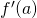is the derivative of a function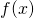in a point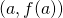and is the slope of the tangent line at that point of the graph of that function.

When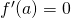it means that the tangent line in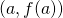has a slope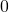and thus that it is horizontal to the-axis. For such a point there are three possibilities:

1. the point is a (local) maximum;
2. the point is a (local) minimum;
3. the point is a horizontal inflection point (an example is the graph of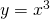).

When we want to know in which points a functionhas a local maximum, minimum or horizontal inflection point, we calculate the derivative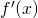and try to find for which values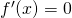, for these values ofthe derivative equals zero and the tangent line is horizontal.

##### Example 1

Determine the minimum of the parabola: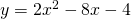We already know that this parabola 'opens up' (the coefficient of the square equals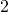and thus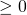) and thus has a minimum that lies on the symmetry-axis, see Quadratic functions and graphs.

We differentiate the function and get: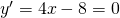and thus: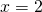The parabola has a minimum in the point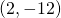.

##### Example 2

Quadratic functions (the graphs are parabolas):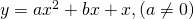have a maximum or minimum that lies on the symmetry-axis: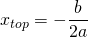We can also calculate this by differentiation. The derivative of the function above is: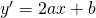The derivative is equal towhen: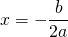which is the-coordinate of the top.

The values offor which the derivative of the function equalsare candidates for a maximum, minimum or horizontal inflection point, but we do not know yet which of the three is the case. This requires further investigation. There are two different methods for this.

Method 1: consider the sign of the first derivative

The derivative of a maximum in a point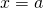is equal to.
Left to the maximum the function is increasing and thus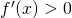for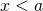.
Right to the maximum the function is decreasing and thusfor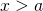.
So the sign of the first derivativearound the maximumhas the following signs:

maximum : +-.

Similarly, the sign of the first derivativearound the minimumhas the following signs:

minimum: -+.

For a horizontal inflection point we find similarly:

horizontal inflection point : ++   or   --.

Method 2: consider the 2nd derivative

Whenis one of the solutions of the equationit is a candidate for a maximum, minimum or horizontal inflection point. Then the following holds.
If: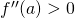, thenis a minimum;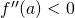, thenis a maximum;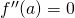, thenis a horizontal inflection point.

##### Example 3

Check whether the function: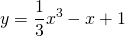has a maximum and/or a minimum.

The derivative is: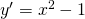The derivative equalsif: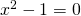so if: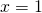or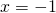The corresponding points on the graph are, respectively: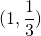or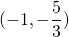Now we check both methods.

Method 1

Aroundwe find the following signs of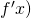:

+- and thus there is a maximum.

Aroundwe find the following signs of:

-+ and thus there is a minimum.

Method 2

We have to calculate the second derivative: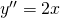Then: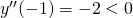and thus the function has a maximum forand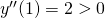and thus the function has a minimum for##### Example 4

Check whether the function: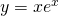has a local maximum or minimum.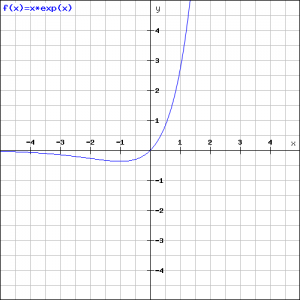The derivative of the function is, using the product rule: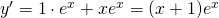The derivative iswhen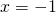and thus the point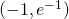is a candidate for either a maximum or minimum. Further research should reveal which of the two it is. It is even possible that it is a point of inflection.
We can use either method, but the second method is more laborious because then we need to use the production rule again. Therefore we take method 1 and only need to consider the sign of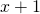because the exponential function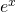is always positive.

If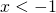thenis negative and ifthenis positief and thus we have a minimum, see the figure.

##### Example 5

Investigate the function:We calculate the derivative: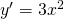The equation: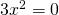has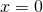as a solution which is a candidate for a maximum, minimum of horizontal inflection point.

The second derivative is: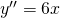which is equal for. So the point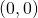is a horizontal inflection point.

0